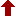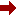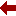#### SLIM21

 Sort Order Chronological Author Title ItemType,Author ItemType,Title Display Format Tabular Publication Bibliography Items / Page 5 10 25

Click the serial number on the left to view the details of the item.
#  AuthorTitleAccn#YearItem Type Claims
1 Nabil Nassif Introduction to computational linear algebra 025999 2015 Book
2 Alan J. Laub Computational matrix analysis 024725 2012 Book
3 S. H. Lui Numerical analysis of partial differential equations 024092 2011 Book
4 HIGHAM, N.J. Accuracy and stability of numerical algorithms 021347 2002 Book
5 Owe Axelsson Iterative solution methods 011166 1994 Book
 (page:1 / 1) [#5]

1.TitleIntroduction to computational linear algebra
Author(s)Nabil Nassif;Jocelyne Erhel;Bernard Philippe
PublicationBoca Raton, CRC Press, 2015.
Descriptionxxiv, 235p.
Abstract NoteBook presents classroom-tested material on computational linear algebra and its application to numerical solutions of partial and ordinary differential equations. The book is designed for senior undergraduate students in mathematics and engineering as well as first-year graduate students in engineering and computational science. The text first introduces BLAS operations of types 1, 2, and 3 adapted to a scientific computer environment, specifically MATLAB. It next covers the basic mathematical tools needed in numerical linear algebra and discusses classical material on Gauss decompositions as well as LU and Choleskys factorizations of matrices. The text then shows how to solve linear least squares problems, provides a detailed numerical treatment of the algebraic eigenvalue problem, and discusses (indirect) iterative methods to solve a system of linear equations. The final chapter illustrates how to solve discretized sparse systems of linear equations. Each chapter ends with exercises and computer projects.
ISBN,Price9781482258691 : UKP 49.99(HB)
Classification512.64:681.3
Keyword(s)1. BLAS OPERATION 2. COMPUTATIONAL LINEAR ALGEBRA 3. KRYLON METHOD 4. LINEAR ALGEBRA 5. MATLAB 6. NUMERICAL LINEAR ALGEBRA
Item TypeBook

Circulation Data
 Accession# Call# Status Issued To Return Due On Physical Location 025999 512.64:681.3/NAS/025999 On Shelf

+Copy Specific Information
2.TitleComputational matrix analysis
Author(s)Alan J. Laub
PublicationPhiladelphia, Society for Industrial and Applied Mathematics, 2012.
Descriptionxiii, 153p.
Abstract NoteThis text provides an introduction to numerical linear algebra together with its application to solving problems arising in state-space control and systems theory. The book provides a number of elements designed to help the reader learn to use numerical linear algebra in day-to-day computing or research, including a brief review of matrix analysis and an introduction to finite (IEEE) arithmetic, alongside discussion of mathematical software topics. In addition to the fundamental concepts, the text covers statistical condition estimation and gives an overview of certain computational problems in control and systems theory. Engineers and scientists will find this text valuable as a theoretical resource to complement their work in algorithms. For graduate students beginning their study, or advanced undergraduates, this text is ideal as a one-semester course in numerical linear algebra and is a natural follow-on to the author's previous book, Matrix Analysis for Scientists and Engineers.
ISBN,Price9781611972207 : UKP 30.00(PB)
Classification512.64:681.3
Keyword(s)1. MATRIX ANALYSIS 2. NUMERICAL LINEAR ALGEBRA
Item TypeBook

Circulation Data
 Accession# Call# Status Issued To Return Due On Physical Location 024725 512.64:681.3/LAUB/024725 On Shelf

+Copy Specific Information
3.TitleNumerical analysis of partial differential equations
Author(s)S. H. Lui
PublicationNew Jersey, John Wiley and Sons, 2011.
Descriptionxiii, 487p.
Abstract NoteNumerical Analysis of Partial Differential Equations provides a comprehensive, self-contained treatment of the quantitative methods used to solve elliptic partial differential equations (PDEs), with a focus on the efficiency as well as the error of the presented methods. The author utilizes coverage of theoretical PDEs, along with the nu merical solution of linear systems and various examples and exercises, to supply readers with an introduction to the essential concepts in the numerical analysis of PDEs. The book presents the three main discretization methods of elliptic PDEs: finite difference, finite elements, and spectral methods. Each topic has its own devoted chapters and is discussed alongside additional key topics, including: The mathematical theory of elliptic PDEs Numerical linear algebra Time-dependent PDEs Multigrid and domain decomposition PDEs posed on infinite domains The book concludes with a discussion of the methods for nonlinear problems, such as Newton's method, and addresses the importance of hands-on work to facilitate learning. Each chapter concludes with a set of exercises, including theoretical and programming problems, that allows readers to test their understanding of the presented theories and techniques. In addition, the book discusses important nonlinear problems in many fields of science and engineering, providing information as to how they can serve as computing projects across various disciplines. Requiring only a preliminary understanding of analysis, Numerical Analysis of Partial Differential Equations is suitable for courses on numerical PDEs at the upper-undergraduate and graduate levels. The book is also appropriate for students majoring in the mathematical sciences and engineering.
ISBN,Price9780470647288 : \$ 114.95(HB)
Classification519.63
Keyword(s)1. ELLIPTIC PARTIAL DIFFERENTIAL 2. NUMERICAL ANALYSIS 3. NUMERICAL LINEAR ALGEBRA 4. PARTIAL DIFFERENTIAL EQUATIONS
Item TypeBook

Circulation Data
 Accession# Call# Status Issued To Return Due On Physical Location 024092 519.63/LUI/024092 On Shelf

+Copy Specific Information
4.TitleAccuracy and stability of numerical algorithms
Author(s)N.J. Higham
Edition2nd.
Description680p.
ISBN,Price0898715210 : US \$67.65
Classification519.6
Keyword(s)1. ARITHMETIC 2. FLOATING POINT ARITHMETIC 3. NONLINEAR SYSTEMS 4. NUMERICAL ANALYSIS - DATA PROCESSING 5. NUMERICAL LINEAR ALGEBRA 6. SKEW-SYMMETRIC SYSTEMS 7. SYMMETRIC INDEFINITE
Item TypeBook

Circulation Data
 Accession# Call# Status Issued To Return Due On Physical Location 021347 519.6/HIG/021347 On Shelf

+Copy Specific Information
5.TitleIterative solution methods
Author(s)Owe Axelsson
PublicationCambridge, Cambridge University Press, 1994.
Descriptionxiii,654pp.
Classification519.612
Keyword(s)1. ITERATIVE METHODS 2. NUMERICAL ANALYSIS 3. NUMERICAL LINEAR ALGEBRA
Item TypeBook

Circulation Data
 Accession# Call# Status Issued To Return Due On Physical Location 011166 519.612/AXE/011166 On Shelf

+Copy Specific Information
 (page:1 / 1) [#5]